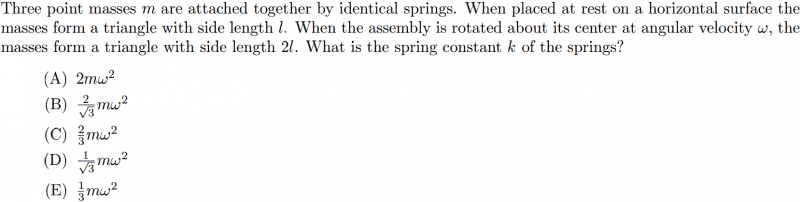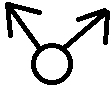# Spring Angular Momentum Problem

• Rmehtany
In summary, the equation for the net inward force is 2*f spring*cos(30)*f centripetal = rad(3) * f spring, and the equation for the mass is k*L*rad(3).f

## Homework Statement## Homework Equations

PE spring = .5 kx^2
KE rotation = .5 I w^2

## The Attempt at a Solution

I tried to do a conservation of energy
(Note: I = moment of inertia, L = length)
3*.5*k L^2 = .5 I w^2

I =3 M R^2 ---> a^2 = b^2 + c^2 -2bc cos(a) (the reason why I am using law of cosines is to find the radius from the center to each point mass)

R = L / sqrt(3)

I = ML^2

3*.5*k*L^2 = .5*M*L^2 *w^2

k = 1/3 * Mw^2 which is wrong. The answer is C. How do you do this?

## Homework Statement

View attachment 77928

## Homework Equations

PE spring = .5 kx^2
KE rotation = .5 I w^2

## The Attempt at a Solution

I tried to do a conservation of energy
(Note: I = moment of inertia, L = length)
3*.5*k L^2 = .5 I w^2

I =3 M R^2 ---> a^2 = b^2 + c^2 -2bc cos(a) (the reason why I am using law of cosines is to find the radius from the center to each point mass)

R = L / sqrt(3)

I = ML^2

3*.5*k*L^2 = .5*M*L^2 *w^2

k = 1/3 * Mw^2 which is wrong. The answer is C. How do you do this?

This is an equilibrium problem. The effective restoring force of the springs, acting on the masses, provides the centripetal force.

I see, Let me try. So the mass is 3m, and the Radius is calculated by law of cosines to be 4L/rad(3). so:

3*k*cos(30)* L = m*w^2 * 4L/rad(3)
k = m*w^2 * 2/9

I'm still messing up. What am I doing?

I see, Let me try. So the mass is 3m, and the Radius is calculated by law of cosines to be 4L/rad(3). so:

3*k*cos(30)* L = m*w^2 * 4L/rad(3)
k = m*w^2 * 2/9

I'm still messing up. What am I doing?
Draw a vector diagram for the forces acting on one of the balls.Set the net inward force provided by the springs equal to the centripetal force, to maintain uniform circular motion.

OK

2 * f spring* cos(30) = f centripetal = rad(3) * f spring